# NCERT Exemplar Class 11 Chemistry Solutions for Chapter 5 - States of Matter

NCERT Exemplar Chemistry Class 11 Chapter 5 States of Matter is available here for free access and download. This study material cover answers to the questions given in NCERT exemplar book, the important questions from previous question papers, states of matter MCQ, states of matter problems and solutions, exercises and worksheets.

By studying this exemplar neatly, students can extract states of matter Class 11 NCERT notes which is very important to save your time during revisions. This notes will come handy when exam nears and you can study the topics in a very short time.

Students are introduced to the topic of states of matter basically in elementary or the primary level. However, as students’ progress into higher and higher classes, the topic gets more advanced and many new terms and concepts are introduced. As such, in Class 11, the topic states of matter is not just knowing about solid liquid or gaseous states but, students have to examine a lot of advance terms like intermolecular forces, liquefaction of gases, thermal interactions, gas laws, kinetic molecular theory, and more. To help students comprehend the different topics easily, free NCERT Exemplar for Class 11 Chemistry Chapter 5 – states of matter is provided here. The exemplar will help students know the topics better and they will also find answers to all the questions given at the end of the chapter. Students who make use of the exemplar can have effective revisions before exams and perform well in the exams.

## Download PDF of NCERT Exemplar Solutions for Class 11 Chemistry Chapter 5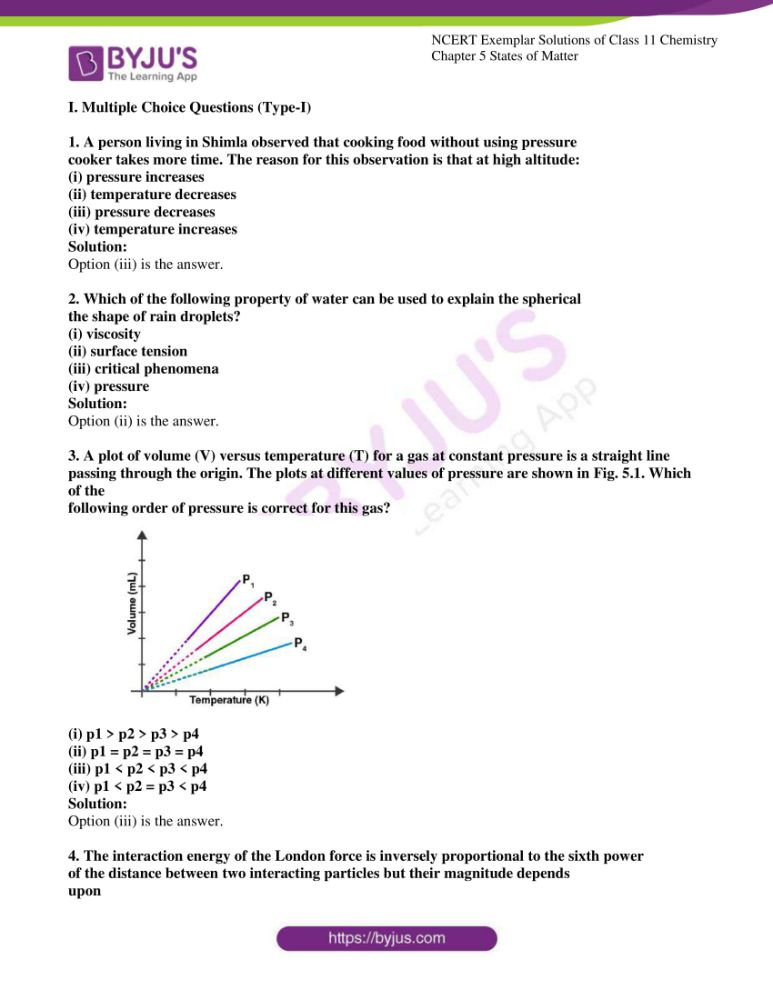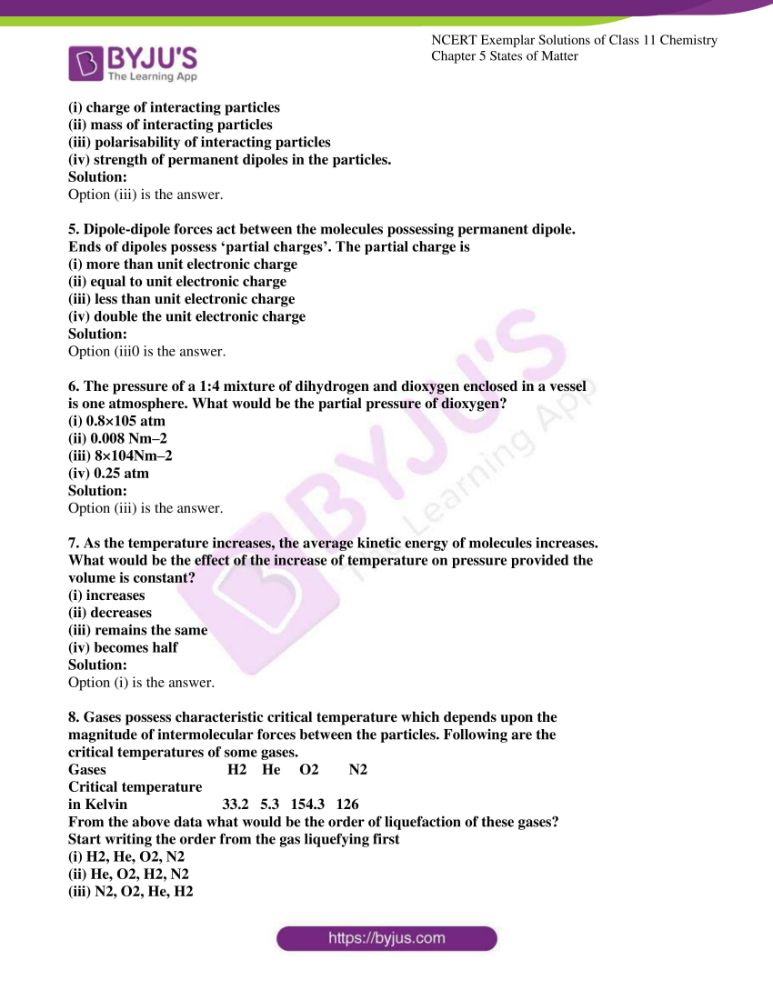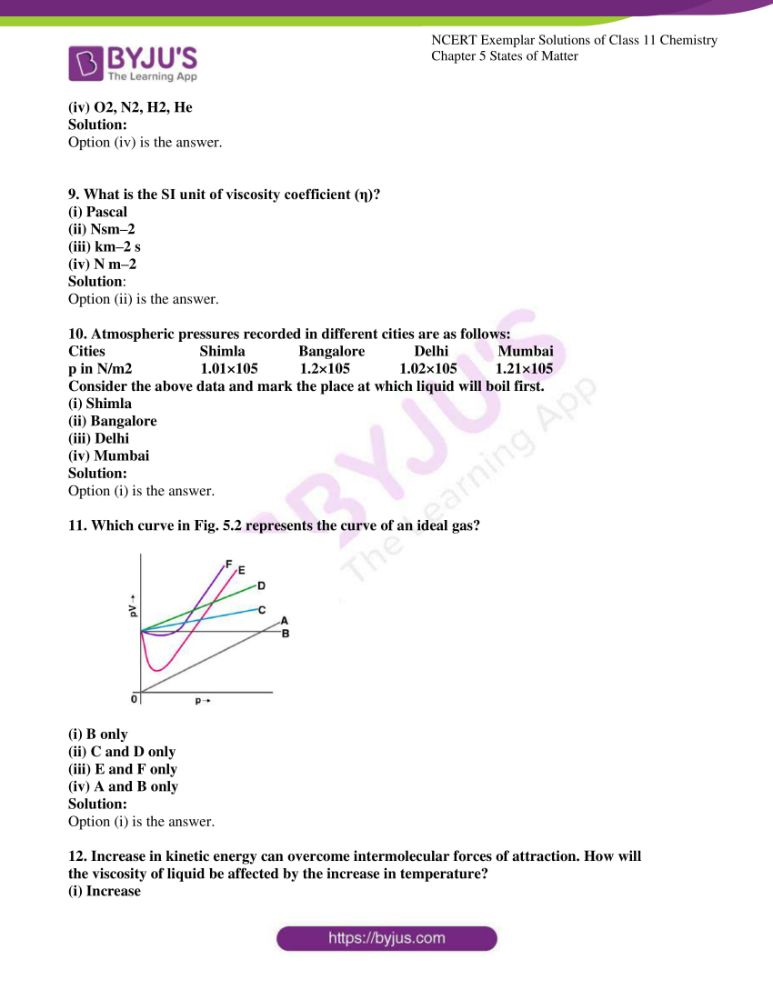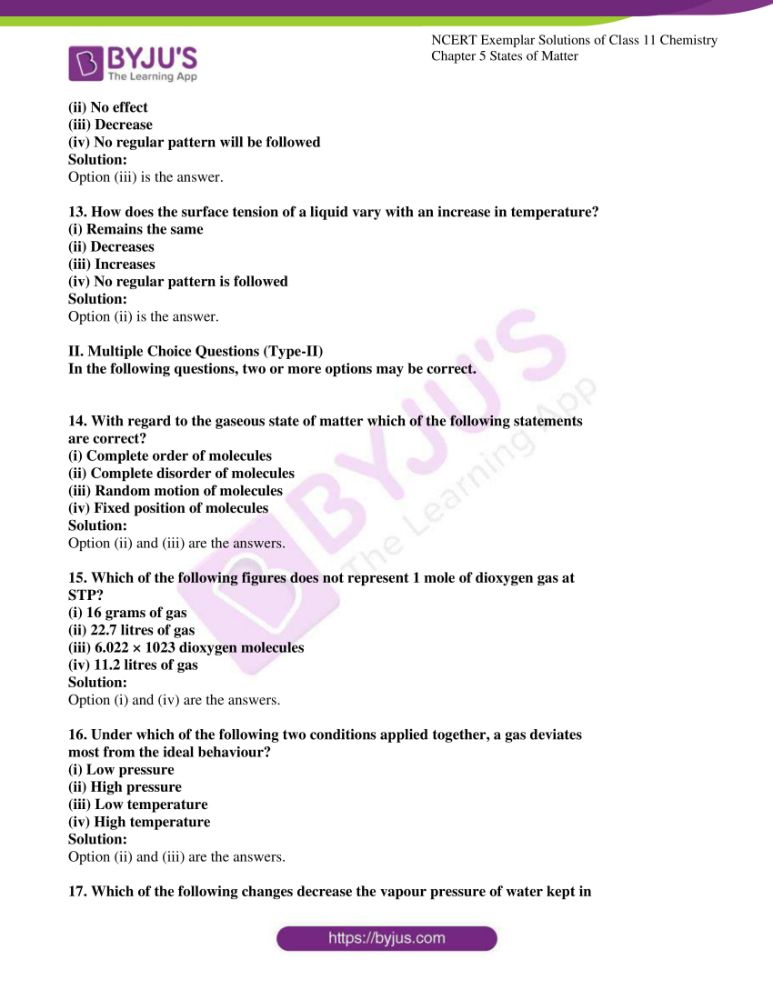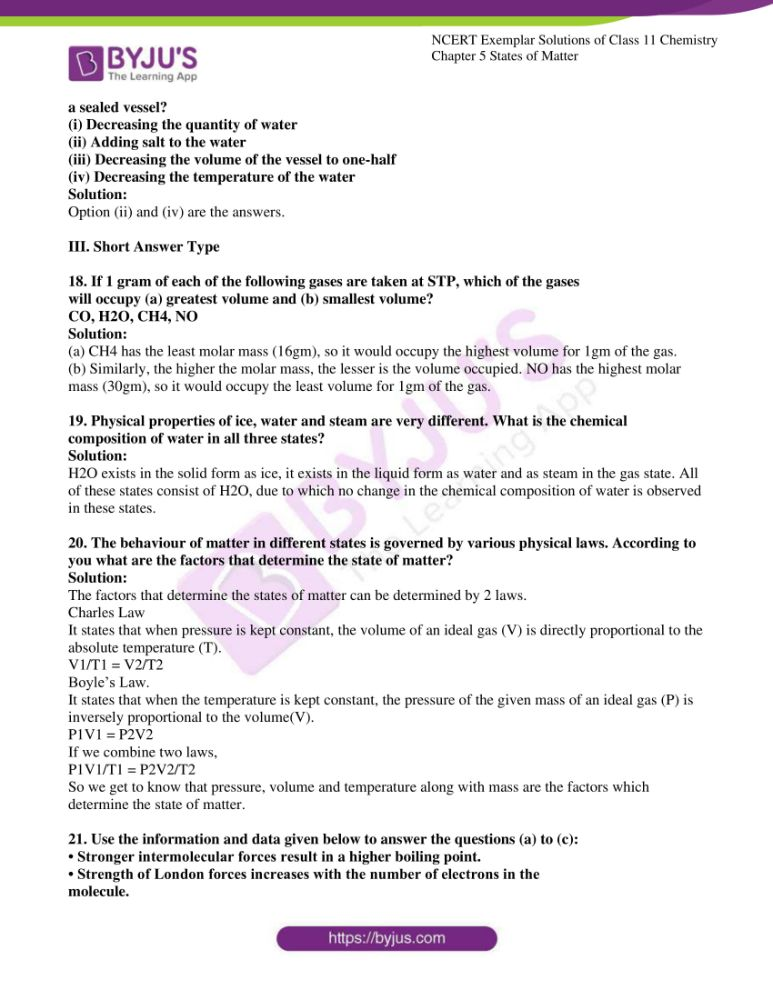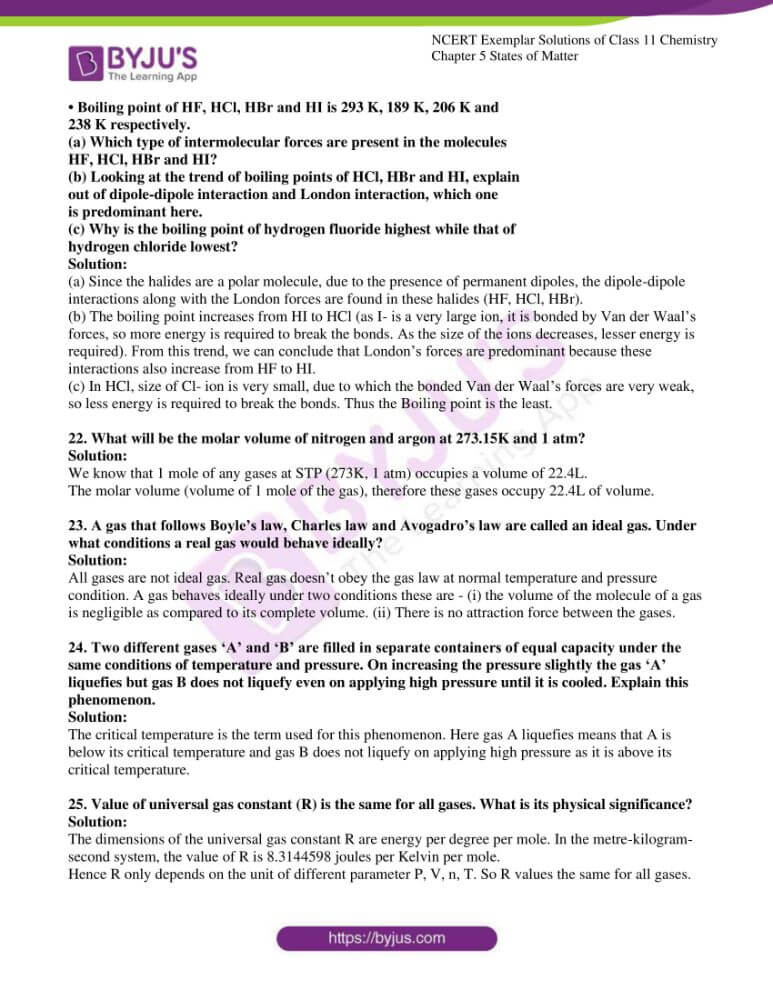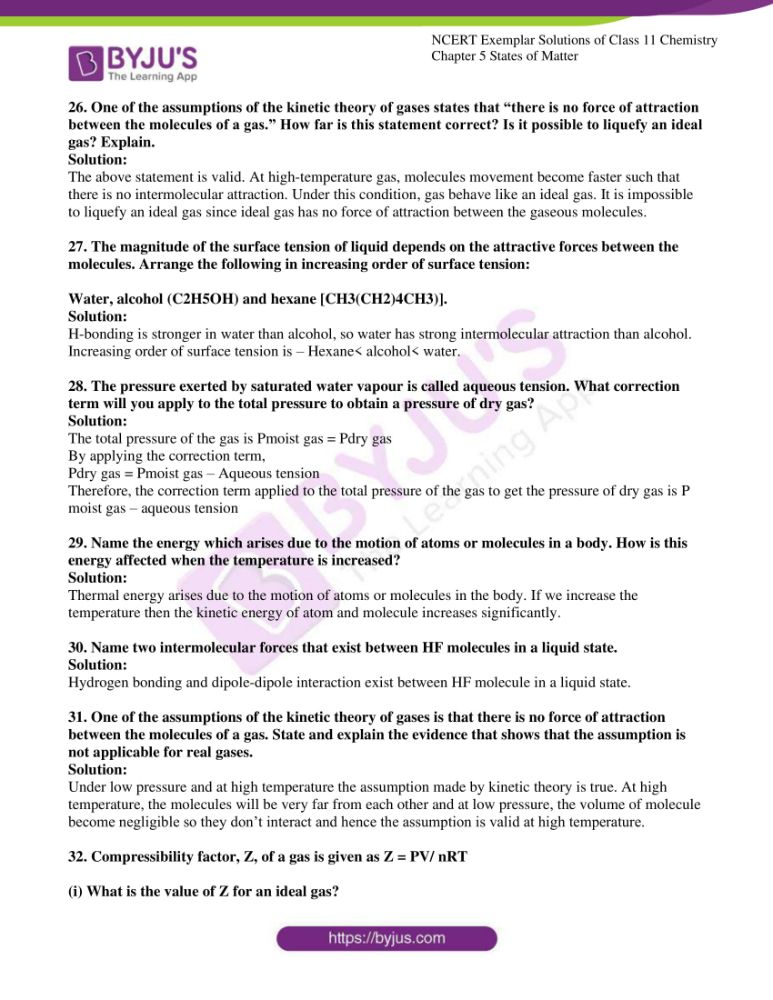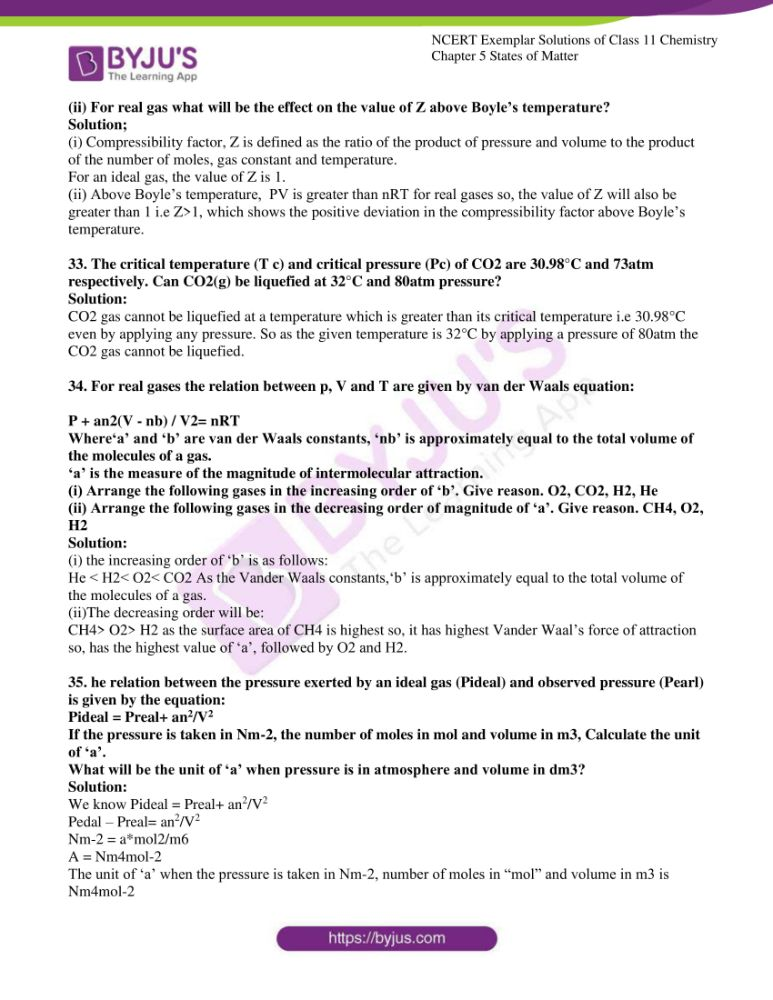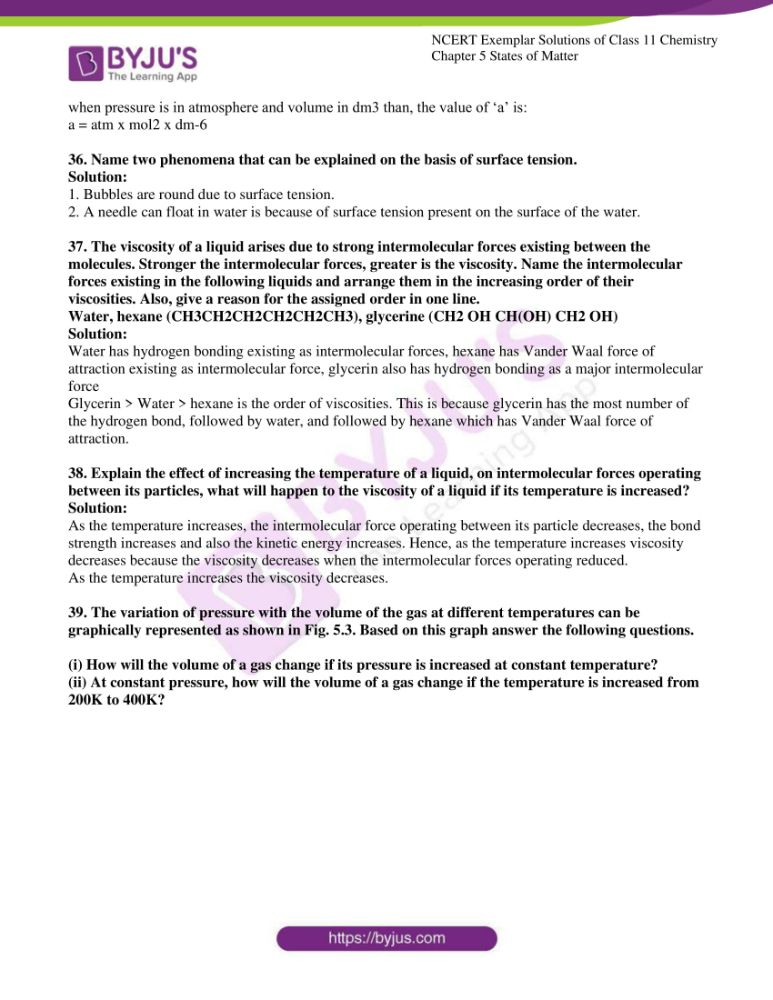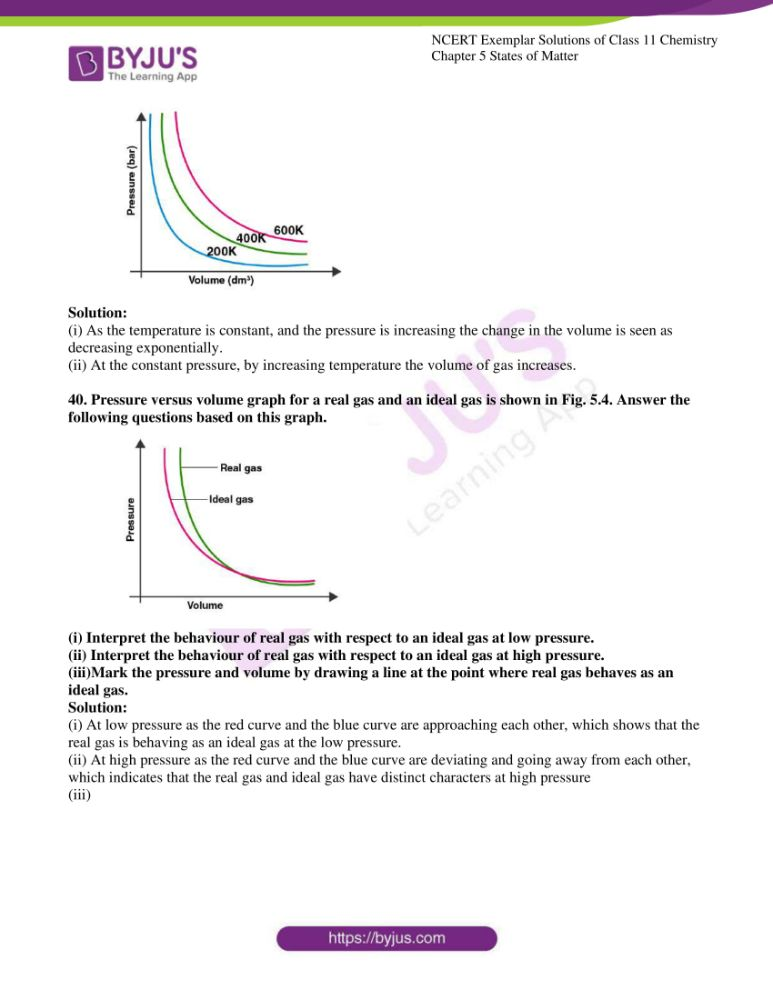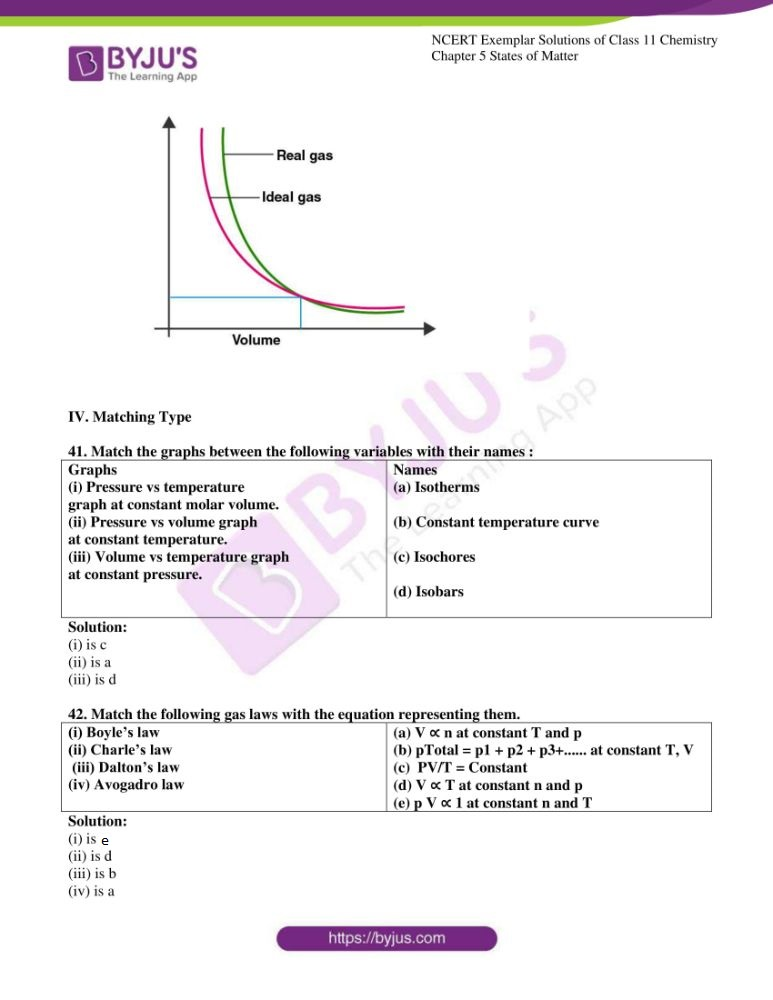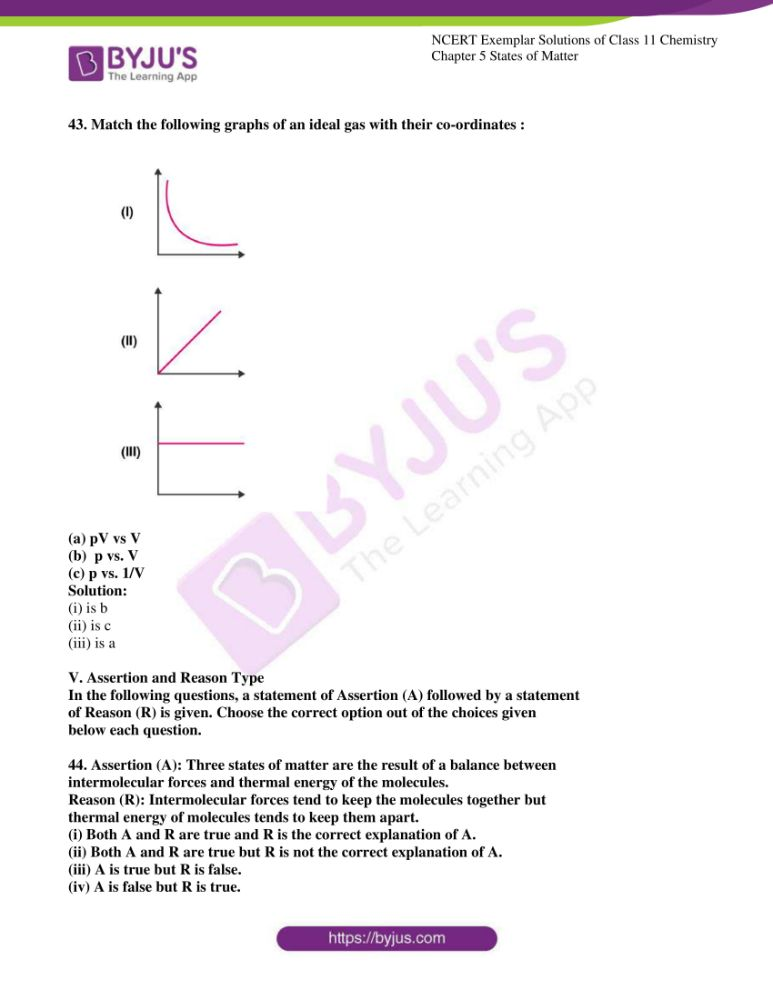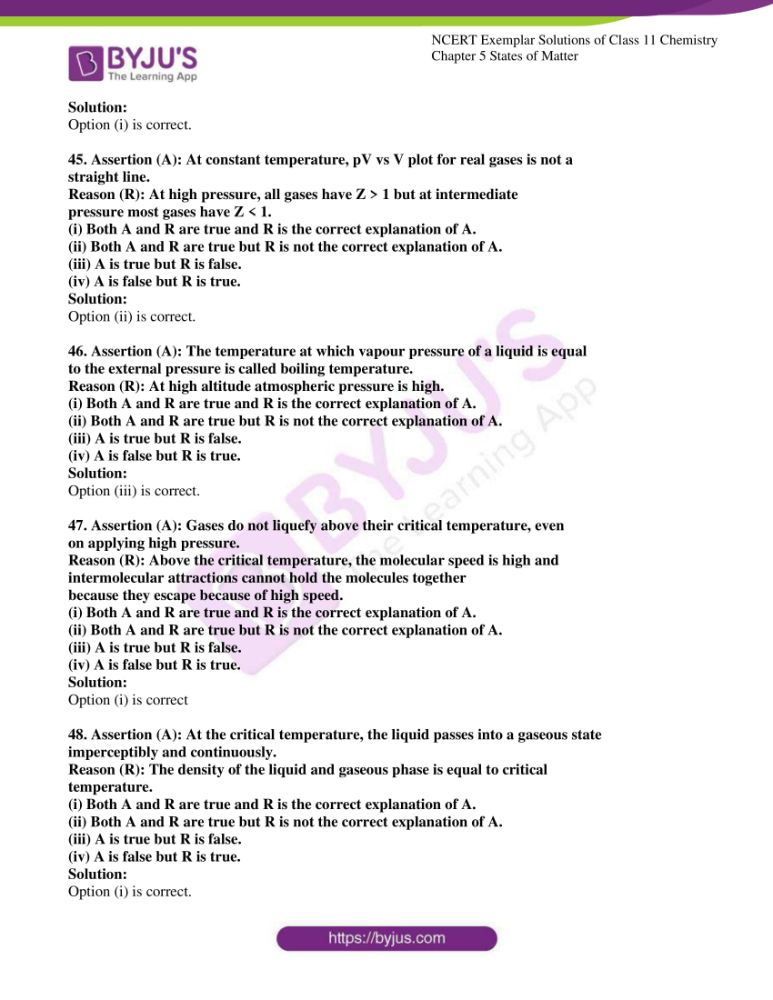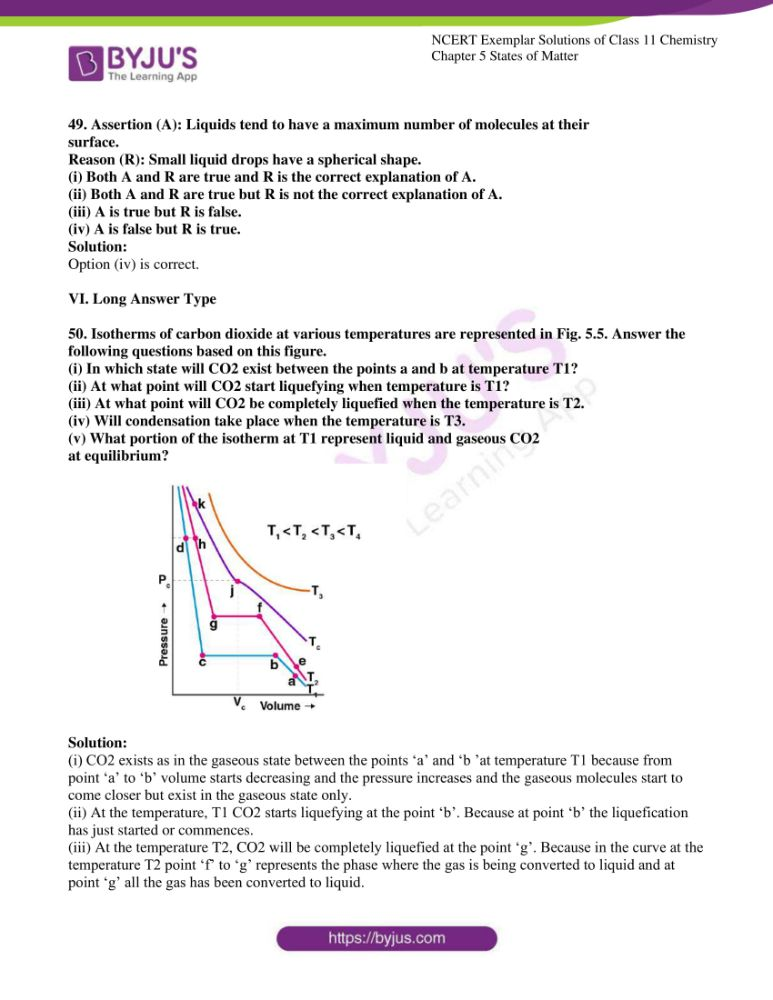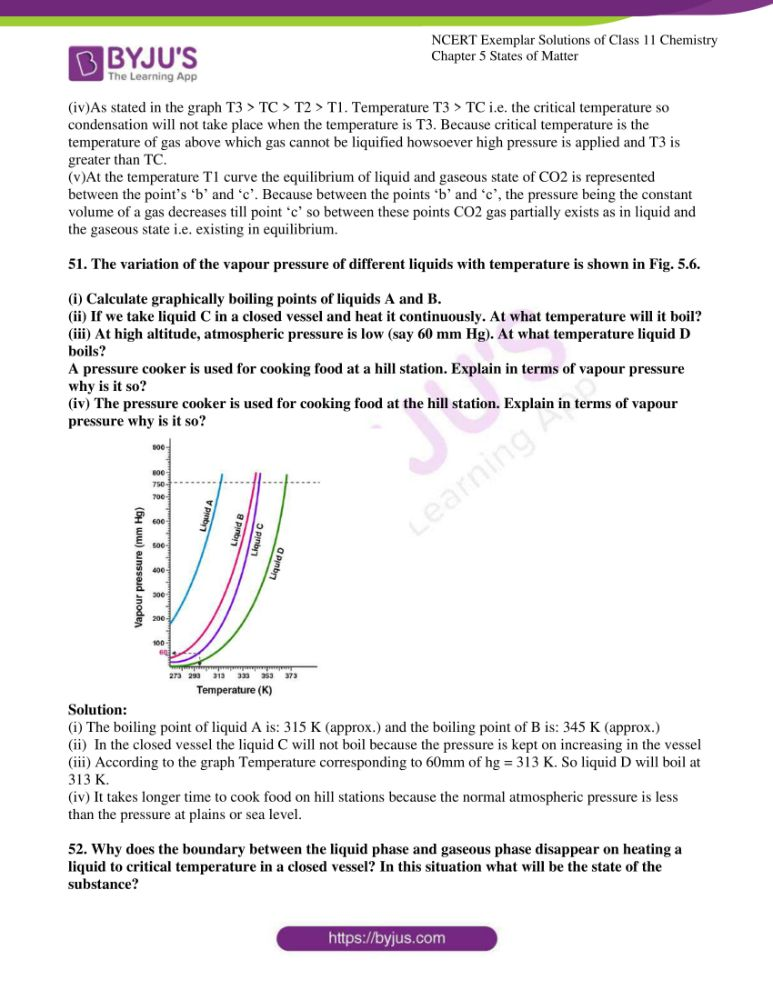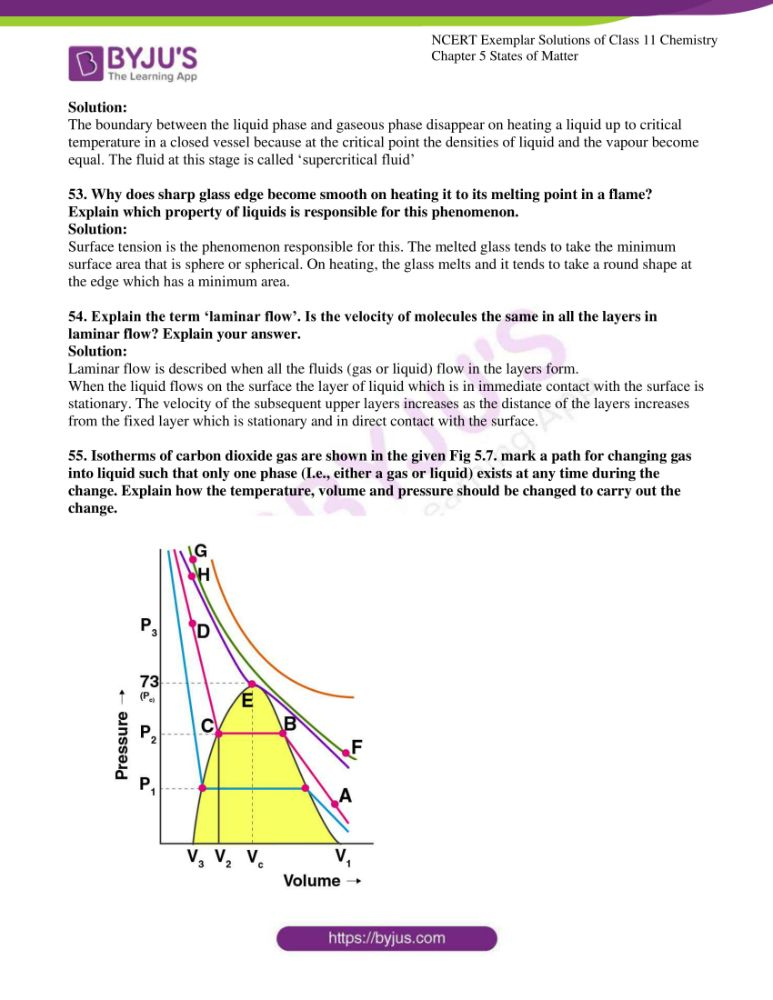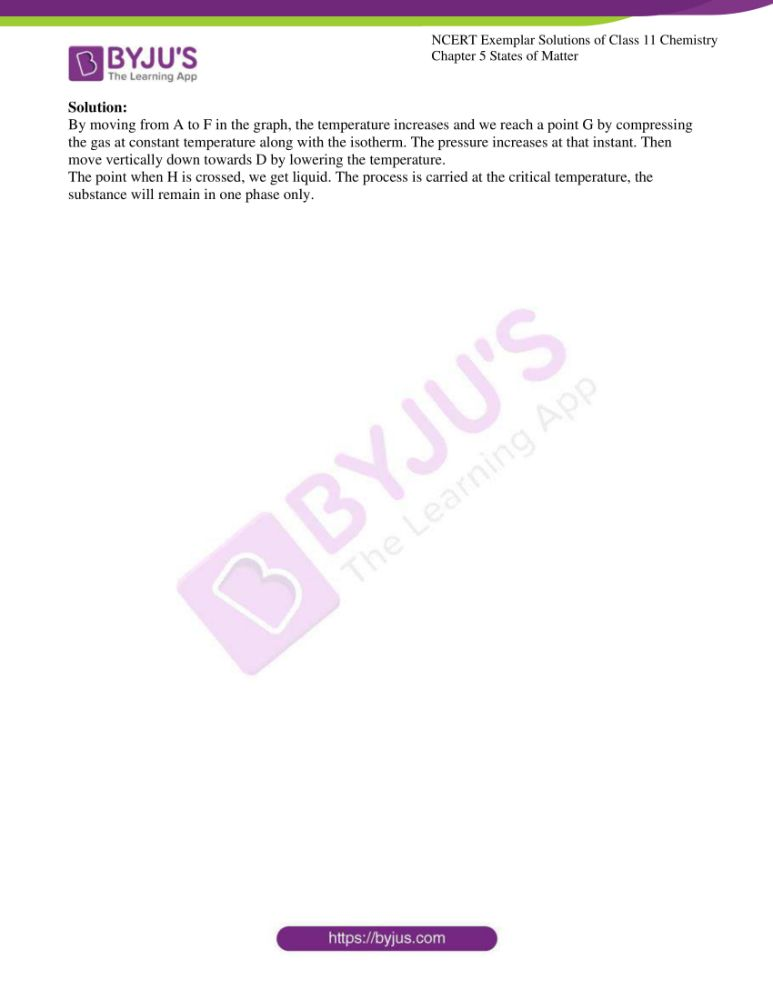### Access Answers to NCERT Exemplar Class 11 Chemistry Chapter 5

I. Multiple Choice Questions (Type-I)

1. A person living in Shimla observed that cooking food without using pressure

cooker takes more time. The reason for this observation is that at high altitude:

(i) pressure increases

(ii) temperature decreases

(iii) pressure decreases

(iv) temperature increases

Solution:

2. Which of the following property of water can be used to explain the spherical

the shape of rain droplets?

(i) viscosity

(ii) surface tension

(iii) critical phenomena

(iv) pressure

Solution:

3. A plot of volume (V) versus temperature (T) for a gas at constant pressure is a straight line passing through the origin. The plots at different values of pressure are shown in Fig. 5.1. Which of the

following order of pressure is correct for this gas?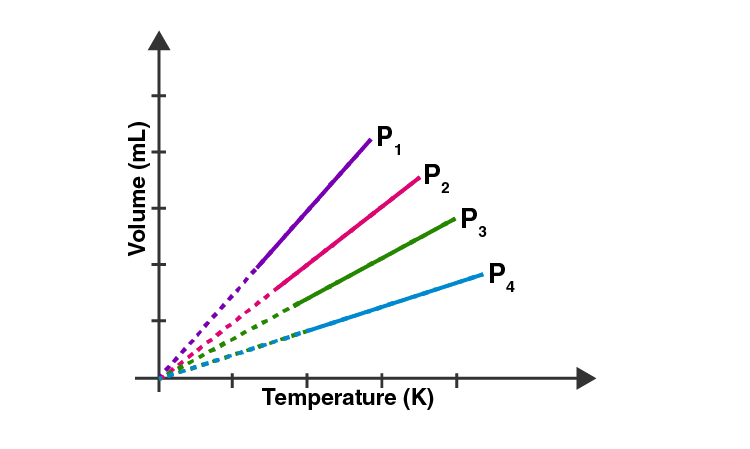(i) p1 > p2 > p3 > p4

(ii) p1 = p2 = p3 = p4

(iii) p1 < p2 < p3 < p4

(iv) p1 < p2 = p3 < p4

Solution:

4. The interaction energy of the London force is inversely proportional to the sixth power

of the distance between two interacting particles but their magnitude depends

upon

(i) charge of interacting particles

(ii) mass of interacting particles

(iii) polarisability of interacting particles

(iv) strength of permanent dipoles in the particles.

Solution:

5. Dipole-dipole forces act between the molecules possessing permanent dipole.

Ends of dipoles possess ‘partial charges’. The partial charge is

(i) more than unit electronic charge

(ii) equal to unit electronic charge

(iii) less than unit electronic charge

(iv) double the unit electronic charge

Solution:

6. The pressure of a 1:4 mixture of dihydrogen and dioxygen enclosed in a vessel

is one atmosphere. What would be the partial pressure of dioxygen?

(i) 0.8×105 atm

(ii) 0.008 Nm–2

(iii) 8×104Nm–2

(iv) 0.25 atm

Solution:

7. As the temperature increases, the average kinetic energy of molecules increases.

What would be the effect of the increase of temperature on pressure provided the

volume is constant?

(i) increases

(ii) decreases

(iii) remains the same

(iv) becomes half

Solution:

8. Gases possess characteristic critical temperature which depends upon the

magnitude of intermolecular forces between the particles. Following are the

critical temperatures of some gases.

Gases H2 He O2 N2

Critical temperature

in Kelvin 33.2 5.3 154.3 126

From the above data what would be the order of liquefaction of these gases?

Start writing the order from the gas liquefying first

(i) H2, He, O2, N2

(ii) He, O2, H2, N2

(iii) N2, O2, He, H2

(iv) O2, N2, H2, He

Solution:

9. What is the SI unit of viscosity coefficient (η)?

(i) Pascal

(ii) Nsm–2

(iii) km–2 s

(iv) N m–2

Solution:

10. Atmospheric pressures recorded in different cities are as follows:

Cities Shimla Bangalore Delhi Mumbai

p in N/m2 1.01×105 1.2×105 1.02×105 1.21×105

Consider the above data and mark the place at which liquid will boil first.

(i) Shimla

(ii) Bangalore

(iii) Delhi

(iv) Mumbai

Solution:

11. Which curve in Fig. 5.2 represents the curve of an ideal gas?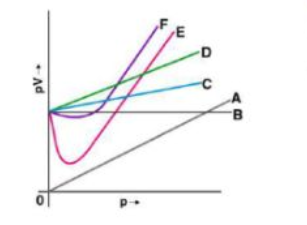(i) B only

(ii) C and D only

(iii) E and F only

(iv) A and B only

Solution:

12. Increase in kinetic energy can overcome intermolecular forces of attraction. How will

the viscosity of liquid be affected by the increase in temperature?

(i) Increase

(ii) No effect

(iii) Decrease

(iv) No regular pattern will be followed

Solution:

13. How does the surface tension of a liquid vary with an increase in temperature?

(i) Remains the same

(ii) Decreases

(iii) Increases

(iv) No regular pattern is followed

Solution:

II. Multiple Choice Questions (Type-II)

In the following questions, two or more options may be correct.

14. With regard to the gaseous state of matter which of the following statements

are correct?

(i) Complete order of molecules

(ii) Complete disorder of molecules

(iii) Random motion of molecules

(iv) Fixed position of molecules

Solution:

Option (ii) and (iii) are the answers.

15. Which of the following figures does not represent 1 mole of dioxygen gas at

STP?

(i) 16 grams of gas

(ii) 22.7 litres of gas

(iii) 6.022 × 1023 dioxygen molecules

(iv) 11.2 litres of gas

Solution:

Option (i) and (iv) are the answers.

16. Under which of the following two conditions applied together, a gas deviates

most from the ideal behaviour?

(i) Low pressure

(ii) High pressure

(iii) Low temperature

(iv) High temperature

Solution:

Option (ii) and (iii) are the answers.

17. Which of the following changes decrease the vapour pressure of water kept in

a sealed vessel?

(i) Decreasing the quantity of water

(ii) Adding salt to the water

(iii) Decreasing the volume of the vessel to one-half

(iv) Decreasing the temperature of the water

Solution:

Option (ii) and (iv) are the answers.

18. If 1 gram of each of the following gases are taken at STP, which of the gases

will occupy (a) greatest volume and (b) smallest volume?

CO, H2O, CH4, NO

Solution:

(a) CH4 has the least molar mass (16gm), so it would occupy the highest volume for 1gm of the gas.

(b) Similarly, the higher the molar mass, the lesser is the volume occupied. NO has the highest molar mass (30gm), so it would occupy the least volume for 1gm of the gas.

19. Physical properties of ice, water and steam are very different. What is the chemical composition of water in all three states?

Solution:

H2O exists in the solid form as ice, it exists in the liquid form as water and as steam in the gas state. All of these states consist of H2O, due to which no change in the chemical composition of water is observed in these states.

20. The behaviour of matter in different states is governed by various physical laws. According to you what are the factors that determine the state of matter?

Solution:

The factors that determine the states of matter can be determined by 2 laws.

Charles Law

It states that when pressure is kept constant, the volume of an ideal gas (V) is directly proportional to the absolute temperature (T).

V1/T1 = V2/T2

Boyle’s Law.

It states that when the temperature is kept constant, the pressure of the given mass of an ideal gas (P) is inversely proportional to the volume(V).

P1V1 = P2V2

If we combine two laws,

P1V1/T1 = P2V2/T2

So we get to know that pressure, volume and temperature along with mass are the factors which determine the state of matter.

21. Use the information and data given below to answer the questions (a) to (c):

• Stronger intermolecular forces result in a higher boiling point.

• Strength of London forces increases with the number of electrons in the

molecule.

• Boiling point of HF, HCl, HBr and HI is 293 K, 189 K, 206 K and

238 K respectively.

(a) Which type of intermolecular forces are present in the molecules

HF, HCl, HBr and HI?

(b) Looking at the trend of boiling points of HCl, HBr and HI, explain

out of dipole-dipole interaction and London interaction, which one

is predominant here.

(c) Why is the boiling point of hydrogen fluoride highest while that of

hydrogen chloride lowest?

Solution:

(a) Since the halides are a polar molecule, due to the presence of permanent dipoles, the dipole-dipole interactions along with the London forces are found in these halides (HF, HCl, HBr).

(b) The boiling point increases from HI to HCl (as I- is a very large ion, it is bonded by Van der Waal’s forces, so more energy is required to break the bonds. As the size of the ions decreases, lesser energy is required). From this trend, we can conclude that London’s forces are predominant because these interactions also increase from HF to HI.

(c) In HCl, size of Cl- ion is very small, due to which the bonded Van der Waal’s forces are very weak, so less energy is required to break the bonds. Thus the Boiling point is the least.

22. What will be the molar volume of nitrogen and argon at 273.15K and 1 atm?

Solution:

We know that 1 mole of any gases at STP (273K, 1 atm) occupies a volume of 22.4L.

The molar volume (volume of 1 mole of the gas), therefore these gases occupy 22.4L of volume.

23. A gas that follows Boyle’s law, Charles law and Avogadro’s law are called an ideal gas. Under what conditions a real gas would behave ideally?

Solution:

All gases are not ideal gas. Real gas doesn’t obey the gas law at normal temperature and pressure condition. A gas behaves ideally under two conditions these are – (i) the volume of the molecule of a gas is negligible as compared to its complete volume. (ii) There is no attraction force between the gases.

24. Two different gases ‘A’ and ‘B’ are filled in separate containers of equal capacity under the same conditions of temperature and pressure. On increasing the pressure slightly the gas ‘A’ liquefies but gas B does not liquefy even on applying high pressure until it is cooled. Explain this phenomenon.

Solution:

The critical temperature is the term used for this phenomenon. Here gas A liquefies means that A is below its critical temperature and gas B does not liquefy on applying high pressure as it is above its critical temperature.

25. Value of universal gas constant (R) is the same for all gases. What is its physical significance?

Solution:

The dimensions of the universal gas constant R are energy per degree per mole. In the metre-kilogram-second system, the value of R is 8.3144598 joules per Kelvin per mole.

Hence R only depends on the unit of different parameter P, V, n, T. So R values the same for all gases.

26. One of the assumptions of the kinetic theory of gases states that “there is no force of attraction between the molecules of a gas.” How far is this statement correct? Is it possible to liquefy an ideal gas? Explain.

Solution:

The above statement is valid. At high-temperature gas, molecules movement become faster such that there is no intermolecular attraction. Under this condition, gas behave like an ideal gas. It is impossible to liquefy an ideal gas since ideal gas has no force of attraction between the gaseous molecules.

27. The magnitude of the surface tension of liquid depends on the attractive forces between the molecules. Arrange the following in increasing order of surface tension:

Water, alcohol (C2H5OH) and hexane [CH3(CH2)4CH3)].

Solution:

H-bonding is stronger in water than alcohol, so water has strong intermolecular attraction than alcohol. Increasing order of surface tension is – Hexane< alcohol< water.

28. The pressure exerted by saturated water vapour is called aqueous tension. What correction term will you apply to the total pressure to obtain a pressure of dry gas?

Solution:

The total pressure of the gas is Pmoist gas = Pdry gas

By applying the correction term,

Pdry gas = Pmoist gas – Aqueous tension

Therefore, the correction term applied to the total pressure of the gas to get the pressure of dry gas is P moist gas – aqueous tension

29. Name the energy which arises due to the motion of atoms or molecules in a body. How is this energy affected when the temperature is increased?

Solution:

Thermal energy arises due to the motion of atoms or molecules in the body. If we increase the temperature then the kinetic energy of atom and molecule increases significantly.

30. Name two intermolecular forces that exist between HF molecules in a liquid state.

Solution:

Hydrogen bonding and dipole-dipole interaction exist between HF molecule in a liquid state.

31. One of the assumptions of the kinetic theory of gases is that there is no force of attraction between the molecules of a gas. State and explain the evidence that shows that the assumption is not applicable for real gases.

Solution:

Under low pressure and at high temperature the assumption made by kinetic theory is true. At high temperature, the molecules will be very far from each other and at low pressure, the volume of molecule become negligible so they don’t interact and hence the assumption is valid at high temperature.

32. Compressibility factor, Z, of a gas is given as Z = PV/ nRT

(i) What is the value of Z for an ideal gas?

(ii) For real gas what will be the effect on the value of Z above Boyle’s temperature?

Solution;

(i) Compressibility factor, Z is defined as the ratio of the product of pressure and volume to the product of the number of moles, gas constant and temperature.

For an ideal gas, the value of Z is 1.

(ii) Above Boyle’s temperature, PV is greater than nRT for real gases so, the value of Z will also be greater than 1 i.e Z>1, which shows the positive deviation in the compressibility factor above Boyle’s temperature.

33. The critical temperature (T c) and critical pressure (Pc) of CO2 are 30.98°C and 73atm respectively. Can CO2(g) be liquefied at 32°C and 80atm pressure?

Solution:

CO2 gas cannot be liquefied at a temperature which is greater than its critical temperature i.e 30.98°C even by applying any pressure. So as the given temperature is 32°C by applying a pressure of 80atm the CO2 gas cannot be liquefied.

34. For real gases the relation between p, V and T are given by van der Waals equation:

P + an2(V – nb) / V2= nRT

Where‘a’ and ‘b’ are van der Waals constants, ‘nb’ is approximately equal to the total volume of the molecules of a gas.

‘a’ is the measure of the magnitude of intermolecular attraction.

(i) Arrange the following gases in the increasing order of ‘b’. Give reason. O2, CO2, H2, He

(ii) Arrange the following gases in the decreasing order of magnitude of ‘a’. Give reason. CH4, O2, H2

Solution:

(i) the increasing order of ‘b’ is as follows:

He < H2< O2< CO2 As the Vander Waals constants,‘b’ is approximately equal to the total volume of the molecules of a gas.

(ii)The decreasing order will be:

CH4> O2> H2 as the surface area of CH4 is highest so, it has highest Vander Waal’s force of attraction so, has the highest value of ‘a’, followed by O2 and H2.

35. he relation between the pressure exerted by an ideal gas (Pideal) and observed pressure (Pearl) is given by the equation:

Pideal = Preal+ an2/V2

If the pressure is taken in Nm-2, the number of moles in mol and volume in m3, Calculate the unit of ‘a’.

What will be the unit of ‘a’ when pressure is in atmosphere and volume in dm3?

Solution:

We know Pideal = Preal+ an2/V2

Pedal – Preal= an2/V2

Nm-2 = a*mol2/m6

A = Nm4mol-2

The unit of ‘a’ when the pressure is taken in Nm-2, number of moles in “mol” and volume in m3 is Nm4mol-2

when pressure is in atmosphere and volume in dm3 than, the value of ‘a’ is:

a = atm x mol2 x dm-6

36. Name two phenomena that can be explained on the basis of surface tension.

Solution:

1. Bubbles are round due to surface tension.

2. A needle can float in water is because of surface tension present on the surface of the water.

37. The viscosity of a liquid arises due to strong intermolecular forces existing between the molecules. Stronger the intermolecular forces, greater is the viscosity. Name the intermolecular forces existing in the following liquids and arrange them in the increasing order of their viscosities. Also, give a reason for the assigned order in one line.

Water, hexane (CH3CH2CH2CH2CH2CH3), glycerine (CH2 OH CH(OH) CH2 OH)

Solution:

Water has hydrogen bonding existing as intermolecular forces, hexane has Vander Waal force of attraction existing as intermolecular force, glycerin also has hydrogen bonding as a major intermolecular force

Glycerin > Water > hexane is the order of viscosities. This is because glycerin has the most number of the hydrogen bond, followed by water, and followed by hexane which has Vander Waal force of attraction.

38. Explain the effect of increasing the temperature of a liquid, on intermolecular forces operating between its particles, what will happen to the viscosity of a liquid if its temperature is increased?

Solution:

As the temperature increases, the intermolecular force operating between its particle decreases, the bond strength increases and also the kinetic energy increases. Hence, as the temperature increases viscosity decreases because the viscosity decreases when the intermolecular forces operating reduced.

As the temperature increases the viscosity decreases.

39. The variation of pressure with the volume of the gas at different temperatures can be graphically represented as shown in Fig. 5.3. Based on this graph answer the following questions.

(i) How will the volume of a gas change if its pressure is increased at constant temperature?

(ii) At constant pressure, how will the volume of a gas change if the temperature is increased from 200K to 400K?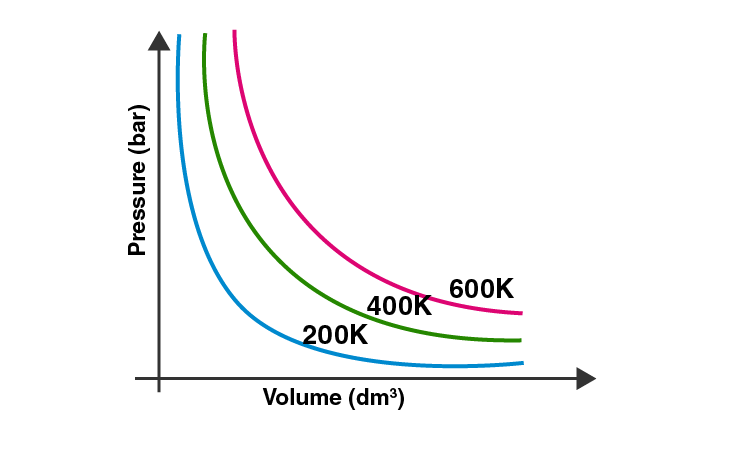Solution:

(i) As the temperature is constant, and the pressure is increasing the change in the volume is seen as decreasing exponentially.

(ii) At the constant pressure, by increasing temperature the volume of gas increases.

40. Pressure versus volume graph for a real gas and an ideal gas is shown in Fig. 5.4. Answer the following questions based on this graph.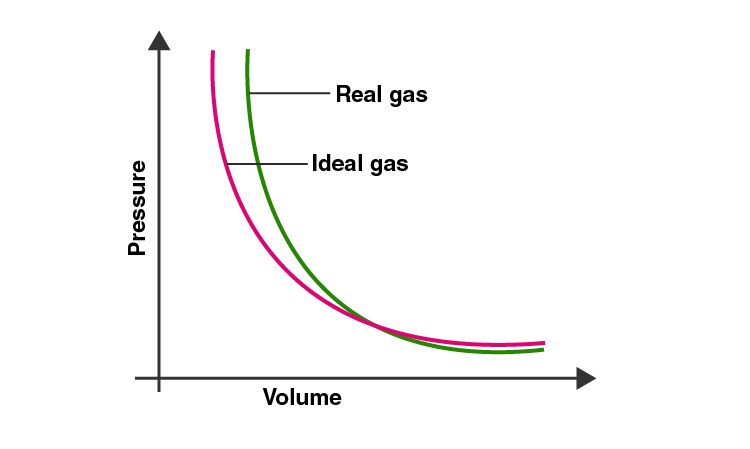(i) Interpret the behaviour of real gas with respect to an ideal gas at low pressure.

(ii) Interpret the behaviour of real gas with respect to an ideal gas at high pressure.

(iii)Mark the pressure and volume by drawing a line at the point where real gas behaves as an ideal gas.

Solution:

(i) At low pressure as the red curve and the blue curve are approaching each other, which shows that the real gas is behaving as an ideal gas at the low pressure.

(ii) At high pressure as the red curve and the blue curve are deviating and going away from each other, which indicates that the real gas and ideal gas have distinct characters at high pressure

(iii)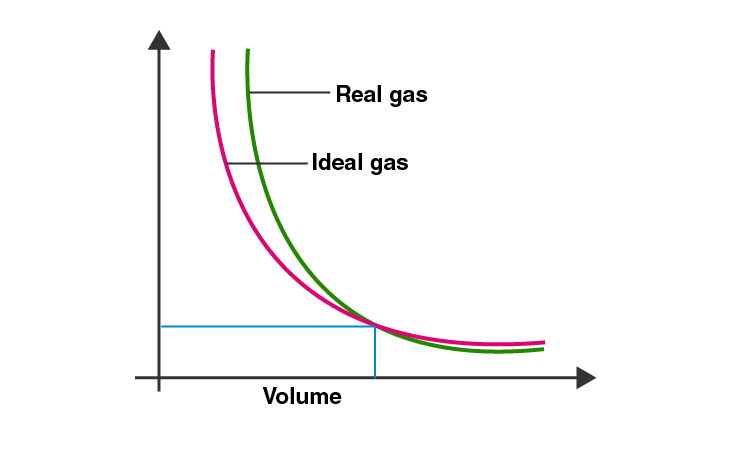IV. Matching Type

41. Match the graphs between the following variables with their names :

 Graphs (i) Pressure vs temperature graph at constant molar volume. (ii) Pressure vs volume graph at constant temperature. (iii) Volume vs temperature graph at constant pressure. Names (a) Isotherms (b) Constant temperature curve (c) Isochores (d) Isobars

Solution:

(i) is c

(ii) is a

(iii) is d

42. Match the following gas laws with the equation representing them.

 (i) Boyle’s law (ii) Charle’s law (iii) Dalton’s law (iv) Avogadro law (a) V ∝ n at constant T and p (b) pTotal = p1 + p2 + p3+…… at constant T, V (c) PV/T = Constant (d) V ∝ T at constant n and p (e) p V ∝ 1 at constant n and T

Solution:

(i) is e

(ii) is d

(iii) is b

(iv) is a

43. Match the following graphs of an ideal gas with their co-ordinates :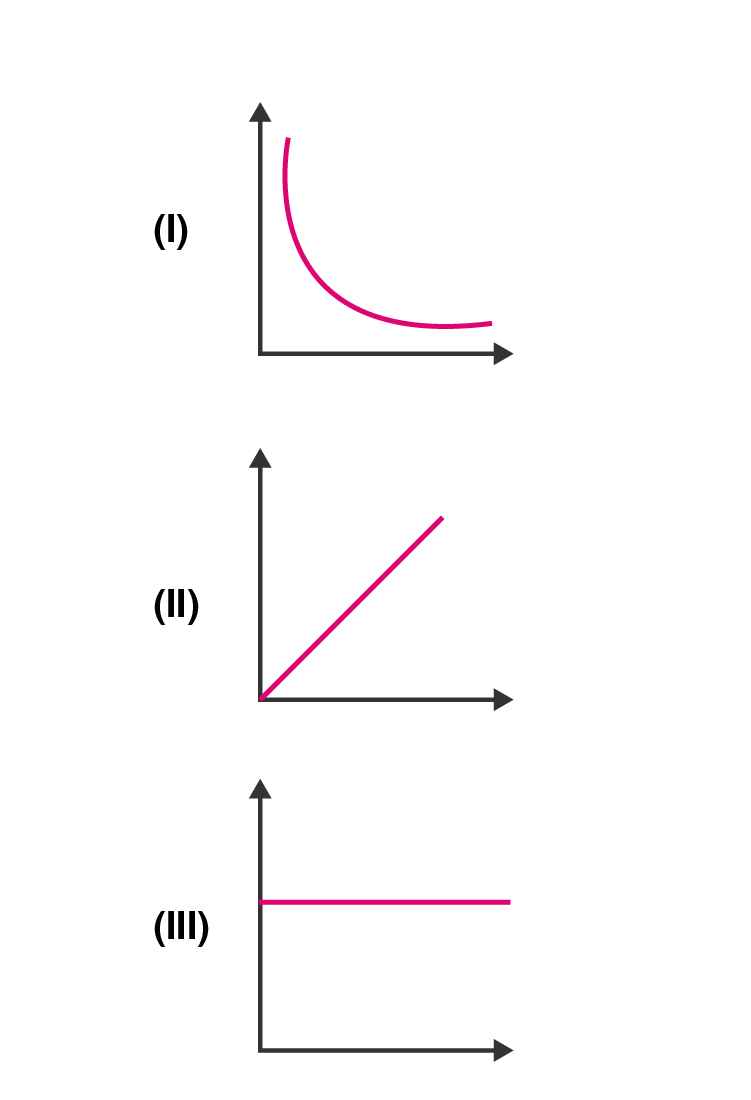(a) pV vs V

(b) p vs. V

(c) p vs. 1/V

Solution:

(i) is b

(ii) is c

(iii) is a

V. Assertion and Reason Type

In the following questions, a statement of Assertion (A) followed by a statement

of Reason (R) is given. Choose the correct option out of the choices given

below each question.

44. Assertion (A): Three states of matter are the result of a balance between

intermolecular forces and thermal energy of the molecules.

Reason (R): Intermolecular forces tend to keep the molecules together but

thermal energy of molecules tends to keep them apart.

(i) Both A and R are true and R is the correct explanation of A.

(ii) Both A and R are true but R is not the correct explanation of A.

(iii) A is true but R is false.

(iv) A is false but R is true.

Solution:

Option (i) is correct.

45. Assertion (A): At constant temperature, pV vs V plot for real gases is not a

straight line.

Reason (R): At high pressure, all gases have Z > 1 but at intermediate

pressure most gases have Z < 1.

(i) Both A and R are true and R is the correct explanation of A.

(ii) Both A and R are true but R is not the correct explanation of A.

(iii) A is true but R is false.

(iv) A is false but R is true.

Solution:

Option (ii) is correct.

46. Assertion (A): The temperature at which vapour pressure of a liquid is equal

to the external pressure is called boiling temperature.

Reason (R): At high altitude atmospheric pressure is high.

(i) Both A and R are true and R is the correct explanation of A.

(ii) Both A and R are true but R is not the correct explanation of A.

(iii) A is true but R is false.

(iv) A is false but R is true.

Solution:

Option (iii) is correct.

47. Assertion (A): Gases do not liquefy above their critical temperature, even

on applying high pressure.

Reason (R): Above the critical temperature, the molecular speed is high and

intermolecular attractions cannot hold the molecules together

because they escape because of high speed.

(i) Both A and R are true and R is the correct explanation of A.

(ii) Both A and R are true but R is not the correct explanation of A.

(iii) A is true but R is false.

(iv) A is false but R is true.

Solution:

Option (i) is correct

48. Assertion (A): At the critical temperature, the liquid passes into a gaseous state

imperceptibly and continuously.

Reason (R): The density of the liquid and gaseous phase is equal to critical

temperature.

(i) Both A and R are true and R is the correct explanation of A.

(ii) Both A and R are true but R is not the correct explanation of A.

(iii) A is true but R is false.

(iv) A is false but R is true.

Solution:

Option (i) is correct.

49. Assertion (A): Liquids tend to have a maximum number of molecules at their

surface.

Reason (R): Small liquid drops have a spherical shape.

(i) Both A and R are true and R is the correct explanation of A.

(ii) Both A and R are true but R is not the correct explanation of A.

(iii) A is true but R is false.

(iv) A is false but R is true.

Solution:

Option (iv) is correct.

50. Isotherms of carbon dioxide at various temperatures are represented in Fig. 5.5. Answer the following questions based on this figure.

(i) In which state will CO2 exist between the points a and b at temperature T1?

(ii) At what point will CO2 start liquefying when temperature is T1?

(iii) At what point will CO2 be completely liquefied when the temperature is T2.

(iv) Will condensation take place when the temperature is T3.

(v) What portion of the isotherm at T1 represent liquid and gaseous CO2

at equilibrium?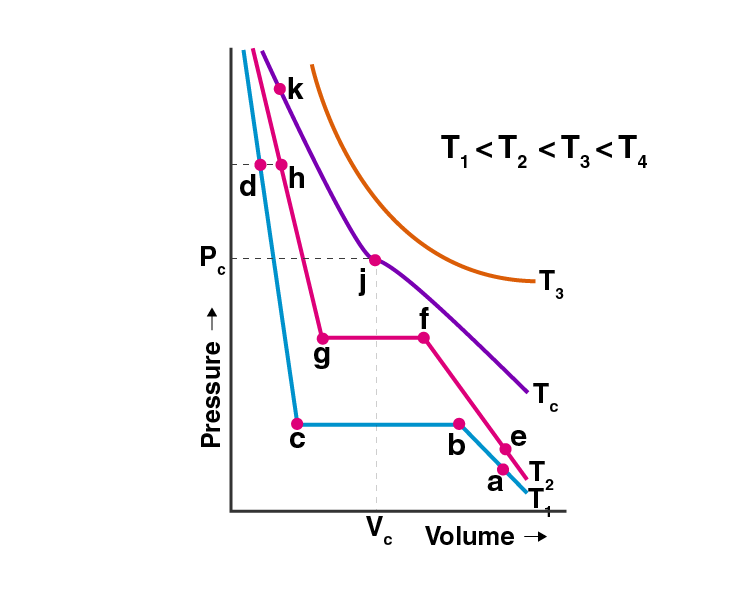Solution:

(i) CO2 exists as in the gaseous state between the points ‘a’ and ‘b ’at temperature T1 because from point ‘a’ to ‘b’ volume starts decreasing and the pressure increases and the gaseous molecules start to come closer but exist in the gaseous state only.

(ii) At the temperature, T1 CO2 starts liquefying at the point ‘b’. Because at point ‘b’ the liquefication has just started or commences.

(iii) At the temperature T2, CO2 will be completely liquefied at the point ‘g’. Because in the curve at the temperature T2 point ‘f’ to ‘g’ represents the phase where the gas is being converted to liquid and at point ‘g’ all the gas has been converted to liquid.

(iv)As stated in the graph T3 > TC > T2 > T1. Temperature T3 > TC i.e. the critical temperature so condensation will not take place when the temperature is T3. Because critical temperature is the temperature of gas above which gas cannot be liquified howsoever high pressure is applied and T3 is greater than TC.

(v)At the temperature T1 curve the equilibrium of liquid and gaseous state of CO2 is represented between the point’s ‘b’ and ‘c’. Because between the points ‘b’ and ‘c’, the pressure being the constant volume of a gas decreases till point ‘c’ so between these points CO2 gas partially exists as in liquid and the gaseous state i.e. existing in equilibrium.

51. The variation of the vapour pressure of different liquids with temperature is shown in Fig. 5.6.

(i) Calculate graphically boiling points of liquids A and B.

(ii) If we take liquid C in a closed vessel and heat it continuously. At what temperature will it boil?

(iii) At high altitude, atmospheric pressure is low (say 60 mm Hg). At what temperature liquid D boils?

A pressure cooker is used for cooking food at a hill station. Explain in terms of vapour pressure why is it so?

(iv) The pressure cooker is used for cooking food at the hill station. Explain in terms of vapour pressure why is it so?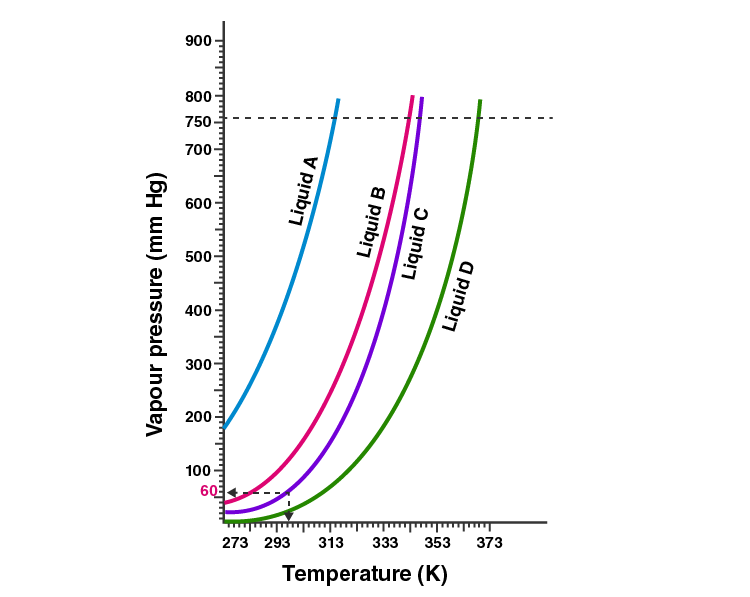Solution:

(i) The boiling point of liquid A is: 315 K (approx.) and the boiling point of B is: 345 K (approx.)

(ii) In the closed vessel the liquid C will not boil because the pressure is kept on increasing in the vessel

(iii) According to the graph Temperature corresponding to 60mm of hg = 313 K. So liquid D will boil at 313 K.

(iv) It takes longer time to cook food on hill stations because the normal atmospheric pressure is less than the pressure at plains or sea level.

52. Why does the boundary between the liquid phase and gaseous phase disappear on heating a liquid to critical temperature in a closed vessel? In this situation what will be the state of the substance?

Solution:

The boundary between the liquid phase and gaseous phase disappear on heating a liquid up to critical temperature in a closed vessel because at the critical point the densities of liquid and the vapour become equal. The fluid at this stage is called ‘supercritical fluid’

53. Why does sharp glass edge become smooth on heating it to its melting point in a flame? Explain which property of liquids is responsible for this phenomenon.

Solution:

Surface tension is the phenomenon responsible for this. The melted glass tends to take the minimum surface area that is sphere or spherical. On heating, the glass melts and it tends to take a round shape at the edge which has a minimum area.

54. Explain the term ‘laminar flow’. Is the velocity of molecules the same in all the layers in laminar flow? Explain your answer.

Solution:

Laminar flow is described when all the fluids (gas or liquid) flow in the layers form.

When the liquid flows on the surface the layer of liquid which is in immediate contact with the surface is stationary. The velocity of the subsequent upper layers increases as the distance of the layers increases from the fixed layer which is stationary and in direct contact with the surface.

55. Isotherms of carbon dioxide gas are shown in the given Fig 5.7. mark a path for changing gas into liquid such that only one phase (I.e., either a gas or liquid) exists at any time during the change. Explain how the temperature, volume and pressure should be changed to carry out the change.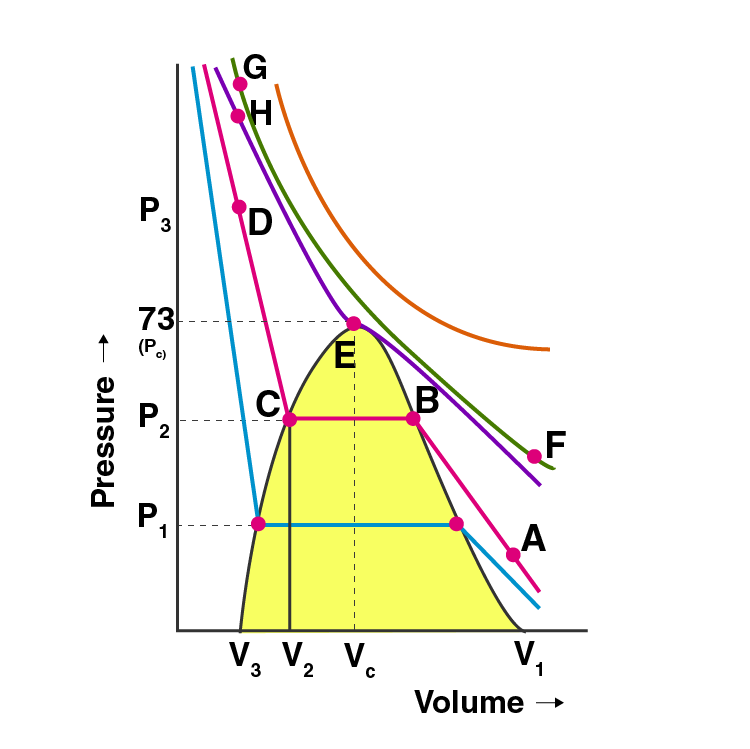Solution:

By moving from A to F in the graph, the temperature increases and we reach a point G by compressing the gas at constant temperature along with the isotherm. The pressure increases at that instant. Then move vertically down towards D by lowering the temperature.

The point when H is crossed, we get liquid. The process is carried at the critical temperature, the substance will remain in one phase only.

#### Important Concepts involved in Class 11 Chemistry Chapter 5 States of Matter: Gases and Liquids

1. Intermolecular Forces
1. Dispersion Forces Or London Forces
2. Dipole-dipole Forces
3. Dipole-induced Dipole Forces
4. Hydrogen Bond
2. Thermal Energy
3. Intermolecular Forces Vs Thermal Interactions
4. The Gaseous State Ex
5. The Gas Laws Ex
1. Boyle’s Law (Pressure-volume Relationship)
2. Charles’ Law (Temperature-volume Relationship)
3. Gay Lussac’s Law (Pressure-temperature Relationship)
4. Avogadro Law (Volume – Amount Relationship)
6. Ideal Gas Equation
1. Density And Molar Mass Of A Gaseous Substance
2. Dalton’s Law Of Partial Pressures
7. Kinetic Molecular Theory Of Gases
8. Behaviour Of Real Gases: Deviation From Ideal Gas Behaviour
9. Liquefaction Of Gases
10. Liquid State
1. Vapour Pressure
2. Surface Tension Ex 5.10.3 – Viscosity.

BYJU’S provides chapter-wise NCERT exemplar solutions for all science subjects of all the classes. In order to score good marks in the examination, students must refer to these NCERT exemplars provided by us.